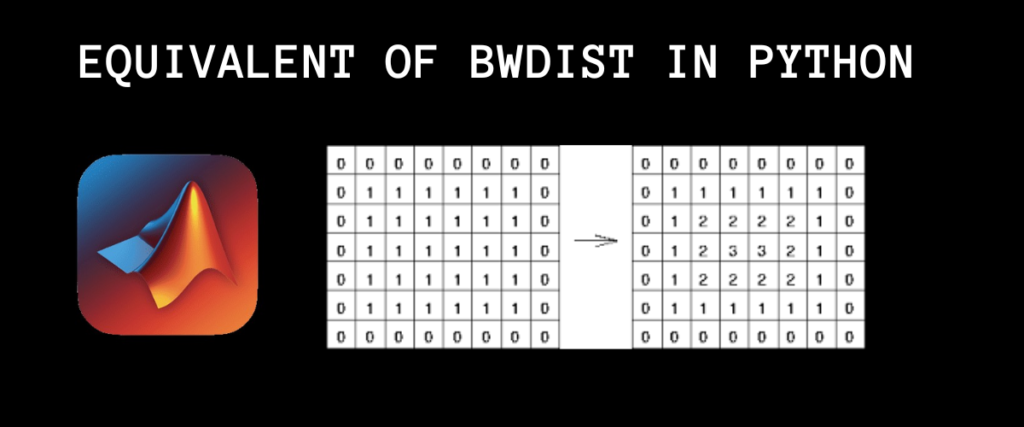# Python Equivalent to Matlab’s Bwdist: A Comprehensive GuideImage Processing is one of the most crucial tasks when dealing with images. It typically means manipulating images like converting the original image to grayscale, computing distance maps, performing segmentation, etc.

One such cumbersome task is finding the distance map or distance transformation between two different regions of an image which will be used to identify patterns, shape matching, and image analysis.

We will look at the Euclidean Distance Transform and how to achieve it using Matlab and Python. Our objective for today is to find a Python equivalent to the bwdist method in Matlab.

Also read: New to Image Processing? Start Here!

## The Euclidean Distance Transformation

We are all familiar with Euclidean Distance since higher grade mathematics where we used this distance metric to compute the distance between two points in the geometry.

Coming to Machine Learning, we use it in the two popular algorithms: KNN and KMeans.

For the unversed, the Euclidean distance metric computes the distance between two points by calculating the difference between corresponding points and returns the value under the root.

The Euclidean distance is inspired by the distance metric. The transformation calculates the shortest distance from each foreground pixel to the nearest background pixel, providing a numerical representation of the image.

Recommended Read: Reduce the image size using Pillow

Let us understand this concept even better. Consider a binary image. We must assume that the background of the image is made up of 0s and the foreground or the main shape in the image is made up of 1s.

For each pixel in the foreground(white region), we calculate the distance between the pixel and the nearest background pixel(the black region).

If we visualize this image in the form of numbers and compute the distance transform, we get the below image(often called a distance map).

Observe the values of the pixels, in the center, the value is 3.16, which is the maximum, making it appear brighter.. The values surrounding the center pixel appear to be translucent as they are close to the value zero. The boundary pixels whose values are 1.0 and 1.41 appear lighter and grayish.

This is the process carried out internally when computing the Euclidean distance transform.

Let us now take a look at the bwdist method of MATLAB.

## The Bwdist Method of MATLAB

To briefly explain the functionality of bwdist, it is used to compute the Euclidean distance transform of the given image as it is or in a pixel format. We are going to see how bwdist works on numbers.

```a = [0, 1, 0, 1; 1, 0, 0, 1; 0, 0, 0, 0; 1, 1, 1, 1];
bin = a > 0;
binMatrix = reshape(bin, 4, 4);
dt = bwdist(binMatrix);
disp('Binary Image:');
disp(binMatrix);
disp('Euclidean Distance Transform:');
disp(dt);
```

In the first line, we defined a 4×4 matrix in the variable a. The image pixels are converted into binary where the values greater than 0 are replaced with 1. The values are reshaped into a matrix in the third line, then the `bwdist` method is applied to the matrix. Lastly, we display the original and transformed images.

## Python Equivalent of Bwdist

We cannot say there is a method or function in Python that can do what bwdist does; however, there is a method in the scipy library that can compute Euclidean Distance Transform just like bwdist.

Although there is an equivalent of bwdist to perform Euclidean Distance Transform in python, we cannot always achieve the same results with each of them as the way MATLAB and Python treat pixels or images can differ.

### The Distance Transform Edt of Scipy

As the name of this method justifies, we can compute the Euclidean distance transform for an image or an image matrix.

```import numpy as np
from scipy.ndimage import distance_transform_edt
binary_image = np.array([[0,1,0,1],
[1,0,0,1],
[0,0,0,0],
[1,1,1,1],
])

distance_transform = distance_transform_edt(binary_image==0)
print("Original image")
print(binary_image)
print("Euclidean Distance Transform:")
print(distance_transform)
```

We import the numpy library and the distance_transform_edt method from the scipy library in the first two lines. We then create a numpy array of values that we used in MATLAB. The fourth line shows the use of the `distance_transform_edt` method. The results of this method are kept in distance_transform. Finally, the original image pixels and the transformed pixels are printed onto the screen.

Notice how there is a slight difference in the last two rows? This is what we were referring to how the languages treat pixels.

But what if we take the array or matrix generated by MATLAB after converting the original array into binary? (Refer to the MATLAB code: `bin = a>0`).

```import numpy as np
from scipy.ndimage import distance_transform_edt
binary_image = np.array([[0,1,0,1],
[1,0,0,1],
[0,0,0,1],
[1,1,0,1],
])

distance_transform = distance_transform_edt(binary_image==0)
print("Original image")
print(binary_image)
print("Euclidean Distance Transform:")
print(distance_transform)
```

We didn’t make any changes to the existing code except we modified the array according to the binary array we got after conversion.

And now we have the same result from both approaches!

## Summary

The distance transformation of an image can be helpful in observing patterns, matching shapes, and image segmentation and acts as a prerequisite in complex algorithms like the Watershed algorithm.

Through this post, we have discussed a distance transformation metric – The Euclidean distance transform and how we can compute the EDT in MATLAB and Python.

The bwdist is a MATLAB method that computes distance transform and the Scipy library of Python can be used to achieve the same computation.

While Matlab and Python may handle image processing differently, both offer powerful tools for distance transformation. Ready to dive deeper into image analysis?